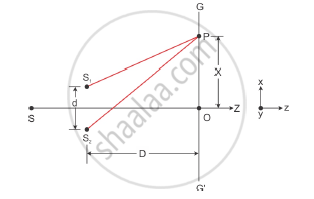Share

# In Young’S Double Slit Experiment, Deduce the Conditions for Obtaining Constructive and Destructive Interference Fringes. - CBSE (Science) Class 12 - Physics

ConceptInterference of Light Waves and Young’S Experiment

#### Question

In young’s double slit experiment, deduce the conditions for obtaining constructive and destructive interference fringes. Hence, deduce the expression for the fringe width.

#### Solution

Young’s double slit experiment demonstrated the phenomenon of interference of light. Consider two fine slits S1 and S2 at a small distance d apart. Let the slits be illuminated by a monochromatic source of light of wavelength λ. Let GG′ be a screen kept at a distance D from the slits. The two waves emanating from slits S1 and S2 superimpose on each other resulting in the formation of an interference pattern on the screen placed parallel to the slits.

Let O be the centre of the distance between the slits. The intensity of light at a point on the screen will depend on the path difference between the two waves reaching that point. Consider an arbitrary point P at a distance x from O on the screen.

Path difference between two waves at P = S2P − S1PThe intensity at the point P is maximum or minimum as the path difference is an integral multiple of wavelength or an odd integral multiple of half wavelength

For the point P to correspond to maxima, we must have

S2P − S1P = n, n = 0, 1, 2, 3...

From the figure given above

(S_2P)^2-(S_1P)^2=D^2+(x+d/2)^2-D^2+(x-d/2)^2

On solving we get:

(S2P)2-(S1P)2=2xd

S_2P-S_1P=(2xd)/(S_2P+S_1P)

As d<<D, then S2P + S2P = 2D  (∵ S1P = S2P ≡ D when d<<D)

:.S_2P-S_1P=(2xd)/(2D)=(xd)/D

Path difference, S_2P-S_1P=(xd)/D

Hence, when constructive interfernce occur, bright region is formed.

For maxima or bright fringe, path difference = xd/D=nlambda

i.e x=(nlambdaD)/d

where n=0,± 1, ±2,........

During destructive interference, dark fringes are formed:

Path difference, (xd)/D=(n+1/2)lambda

x=(n+1/2)(lambdaD)/d

The dark fringe and the bright fringe are equally spaced and the distance between consecutive bright and dark fringe is given by:

β = xn+1-xn

beta=((n+1)lambdaD)/d-(nlambdaD)/d

beta=(lambdaD)/d

Hence the fringe width is given by beta = (lambdaD)/d

Is there an error in this question or solution?

#### Video TutorialsVIEW ALL 

Solution In Young’S Double Slit Experiment, Deduce the Conditions for Obtaining Constructive and Destructive Interference Fringes. Concept: Interference of Light Waves and Young’S Experiment.
S